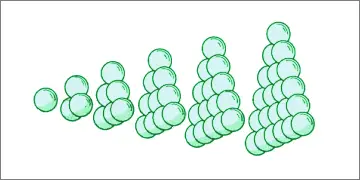# Sum of Consecutive Triangular Numbers & Tetrahedral Numbers

Consecutive Triangular Numbers Sum
Sum of consecutive tri. numbers from
T()  to  T()  inclusive.

The sum of the first n triangular numbers, 1 + 3 + 6 + ... + n(n+1)/2, is called the nth tetrahedral number or triangular pyramidal number. They are so-called because they can be represented by triangular pyramid formations.Rather than add the terms individually to compute the sum of consecutive triangular numbers, you can calculate tetrahedral numbers using the simple summation formula explained below.

### Tetrahedral Number Formula

Let P(n) be the nth triangular pyramidal number. P(n) is defined as the sum

P(n) = 1 + 3 + 6 + 10 + ... + n(n+1)/2 = Σj=1...nT(j).

To find an explicit formula for this sum, we use the fact that the nth square pyramidal number, S(n), is equal to n(n+1)(2n+1)/6. This formula is proved in the square pyramidal number article.

Σj=1...nT(j) = Σj=1...n j(j+1)/2

= Σj/2 + Σj²/2

= (1/2)[Σj + Σj²]

= (1/2)[n(n+1)/2 + n(n+1)(2n+1)/6]

= n(n+1)(n+2)/6

Thus, P(n) = n(n+1)(n+2)/6.

### Sum of Consecutive Triangular Numbers Formula

The sum of any sequence of consecutive triangular numbers from T(a) to T(b) (inclusive) is the difference between the bth tetrahedral number and the ath tetrahedral number plus the ath triangular number. (You need to add T(a) because the sum is inclusive of the endpoints T(a) and T(b).)

Example: Find the sum of the triangular numbers from T(10) to T(20) inclusive.

P(20) - P(10) + T(10)
= 20(21)(22)/6 - 10(11)(12)/6 + 10(11)/2
= 1540 - 220 + 55
= 1375

Notice that this matches the result calculating the sum the long way:

55 + 66 + 78 + 91 + 105 + 120 + 136 + 153 + 181 + 200 + 220 = 1375

### Fun Facts About Tetrahedral Numbers

1. The only tetrahedral numbers that are squares are 1, 4, and 19600.

1² = 1 = P(1)
2² = 4 = P(2)
140² = 19600 = P(49)

2. The only tetrahedral numbers that are also triangular numbers are 1, 10, 120, 1540, and 7140.

T(1) = 1 = P(1)
T(4) = 10 = P(3)
T(15) = 120 = P(8)
T(55) = 1540 = P(20)
T(119) = 7140 = P(34)

3. The height of a stack of spheres in a tetrahedral formation is

d + d(n-1)√2/3,

where d is the diameter of each sphere and n is the number of balls along the bottom edge of the base. See Pyramidal Stack Calculator.

4. The sum of two consecutive tetrahedral numbers, P(n) + P(n-1), is a square pyramidal number, S(n). Proof:

P(n) + P(n-1) = n(n+1)(n+2)/6 + (n-1)n(n+1)/6

= [n³ + 3n² + 2n]/6 + [n³ - n]/6

= [2n³ + 3n² + n]/6

= n(n+1)(2n+1)/6

= S(n)

5. The infinite sum of the reciprocals of tetrahedral numbers is 3/2. This can be shown as follows:

Σn=1...∞1/P(n) = Σn=1...∞6/[n(n+1)(n+2)]

= Σn=1...∞[3/n - 6/(n+1) + 3/(n+2)]

= Σn=1...∞[3/n - 3/(n+1)] - Σn=1...∞[3/(n+1) - 3/(n+2)]

These two sums are telescoping, meaning that consecutive terms in the series cancel out, leaving us with

3 - 3/2 = 3/2.